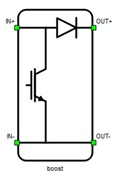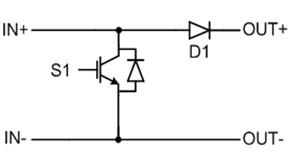# Boost

This section describes boost converters

A block diagram and input parameters for boost converter switching blocks are given in Table 1.

Table 1. The boost converter component in the Schematic Editor core library
component component dialog window component parametersBoost converter• Control (Digital inputs, Internal modulator, Model)
• S1 (1..32)
• S1_logic (active high, active low)
• Gate control enabling (enables usage of external digital signal to enable switching)
• Sen (digital input selection for gate enable)
• Sen_logic (active high, active low)
Weight = 1

A schematic block diagram of the boost switching block is given in Figure 1 with corresponding switch arrangement and naming.

Digital inputs, when selected as the Control parameter, enables you to assign gate drive inputs to any of the digital input pins (from 1 to 32). For example, if S1 is assigned to 1, the digital input pin 1 will be routed to the S1 switch gate drive. In addition, the S1_logic parameter is set to either active high (i.e. high-level input voltage VIH turns on the switch) or active low (i.e. low-level input voltage VIL turns on the switch). The gate drive logic depends on your external controller design.

Internal modulator, when selected as the Control parameter, enables you to use the internal PWM modulator for driving the S1 switch instead of the digital input pins. In this configuration, two additional component inputs will be present. The En input is used to enable/disable PWM modulator control, while In is used as the referent signal input for the internal PWM modulator.

Model, when selected as the Control parameter, enables you to set the IGBT gate drive signal directly from the signal processing model. The input pin gate appears on the component. It is one element input which controls the S1 switch gate. When controlled from the model, logic is always set to active high.Figure 1. Schematic block diagram of boost block diagram with corresponding switch naming

## Losses calculation

When the Losses calculation property is enabled, the component will calculate switching and conduction power losses for all switching elements (IGBTs and diodes). Switching power losses are calculated as a function of current, voltage, and temperature using 3D Look-up tables. 2D input for losses is also supported. When a 2D losses table is inserted, only current and temperature dependance is assumed. Conduction power losses are calculated based on Vce and Rce. Import options and an explanation how to correctly fill all necessary power losses parameters is described in the Importing power losses data section.

Note: An interactive overview of how you can incorporate power loss calculation in your model is available as part of the HIL for Power Electronics course on HIL Academy, as well as in the video Knowledgebase.
Input/output ports for power losses are vectors of two elements. First element (index 0) is IGBT and the second element (index 1) is diode. Available mask properties are:
• Vce - IGBT collector emitter saturation voltage [V]
• Rce - IGBT on state slope resistance [Ohm]
• Vd - Diode voltage drop [V]
• Rd - Diode slope resistance [Ohm]
• Current values - IGBT switching losses, current axis [A]
• Voltage values - IGBT switching losses, voltage axis [V]
• Temp values - IGBT switching losses, temperature axis [°C]
• Et on table out - IGBT switching ON losses, output energy, f(I, V, T) [J]
• Et off table out - IGBT switching OFF losses, output energy, f(I, V, T) [J]
• Ed off table out - Diode switching OFF losses, output energy, f(I, V, T) [J]

## Temperatures calculation

When the Temperatures calculation property is enabled, the component will calculate the combined power losses (P_loss) and junction temperatures (T_junctions) for all switching elements (IGBTs and diodes). Combined power losses represent the sum of the calculated switching and conduction losses transferred through an internally generated Thermal network component. The internally generated Thermal network component also calculates junction temperatures from power losses, input cases temperatures, and provided thermal model parameters. Input/output ports for temperatures calculation are vectors of two elements and they are indexed in the same way as explained in the Losses calculation section.

Note: An interactive overview of how you can incorporate temperature calculation in your model is available as part of the HIL for Power Electronics course on HIL Academy, as well as in the video Knowledgebase.